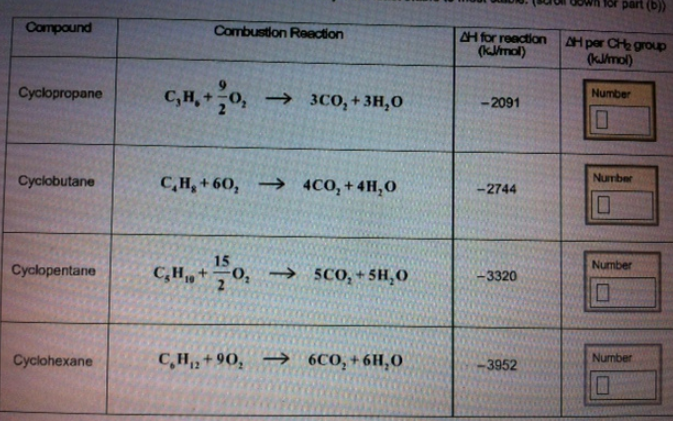# Problem: (a) Given the following reactions and their heats of combustion, calculate the heat of combustion per CH2 for cyclopropane, cyclobutane, cyclopentane and cyclohexane.(b) Rank the four cycloalkanes in order of stability from least stable to most stable.

###### FREE Expert Solution

We simply use the formula below to calculate the heat of combustion per CH2

93% (95 ratings)###### Problem Details

(a) Given the following reactions and their heats of combustion, calculate the heat of combustion per CH2 for cyclopropane, cyclobutane, cyclopentane and cyclohexane.

(b) Rank the four cycloalkanes in order of stability from least stable to most stable.